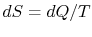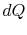Thermodynamics and Propulsion
Subsections

5.2 Axiomatic Statements of the Laws of Thermodynamics

5.2.1 Introduction

As a further aid in familiarization with the second law of thermodynamics and the idea of entropy, we draw an analogy with statements made previously concerning quantities that are closer to experience. In particular, we wish to present once more the Zeroth and First Laws of thermodynamics and use the same framework for the Second Law. In this so-called ``axiomatic formulation,'' the parallels between the Zeroth, First and Second Laws will be made explicit.5.1

5.2.2 Zeroth Law

Section 1.3.2 presented this observation:

Zeroth Law: There exists for every thermodynamic system in equilibrium a property called temperature. Equality of temperature is a necessary and sufficient condition for thermal equilibrium.

The Zeroth law thus defines a property (temperature) and describes its behavior.

5.2.3 First Law

Observations also show that for any system there is a property called the energy. The First Law asserts that one must associate such a property with every system.

First Law: There exists for every thermodynamic system a property called the energy. The change of energy of a system is equal to the mechanical work done on the system in an adiabatic process. In a non-adiabatic process, the change in energy is equal to the heat added to the system minus the mechanical work done by the system.

On the basis of experimental results, therefore, one is led to assert the existence of two new properties, the temperature and internal energy, which do not arise in ordinary mechanics. In a similar way, a further remarkable relationship between heat and temperature will be established, and a new property, the entropy, defined. Although this is a much less familiar property, it is to be stressed that the general approach is quite like that used to establish the Zeroth and First Laws. A general principle and a property associated with any system are extracted from experimental results. Viewed in this way, the entropy should appear no more mystical than the internal energy. The increase of entropy in a naturally occurring process is no less real than the conservation of energy.

5.2.4 Second Law

Although all natural processes must take place in accordance with the First Law, the principle of conservation of energy is, by itself, inadequate for an unambiguous description of the behavior of a system. Specifically, there is no mention of the familiar observation that every natural process has in some sense a preferred direction of action. For example, the flow of heat occurs naturally from hotter to colder bodies, in the absence of other influences, but the reverse flow certainly is not in violation of the First Law. So far as that law is concerned, the initial and final states are symmetrical in a very important respect.

The Second Law is essentially different from the First Law; the two principles are independent and cannot in any sense be deduced from one another. Thus, the concept of energy is not sufficient, and a new property must appear. This property can be developed, and the Second Law introduced, in much the same way as the Zeroth and First Laws were presented. By examination of certain observational results, one attempts to extract from experience a law which is supposed to be general; it is elevated to the position of a fundamental axiom to be proved or disproved by subsequent experiments. Within the structure of classical thermodynamics, there is no proof more fundamental than observations. A statement which can be adopted as the Second Law of thermodynamics is:

Second Law: There exists for every thermodynamic system in equilibrium an extensive scalar property called the entropy,, such that in an infinitesimal reversible change of state of the system,, whereis the absolute temperature andis the amount of heat received by the system. The entropy of a thermally insulated system cannot decrease and is constant if and only if all processes are reversible.

As with the Zeroth and First Laws, the existence of a new property is asserted and its behavior is described.

5.2.5 Reversible Processes

In the course of this development, the idea of a completely reversible process is central, and we can recall the definition, ``a process is called completely reversible if, after the process has occurred, both the system and its surroundings can be wholly restored by any means to their respective initial states'' (first introduced in Section 1.3.3). Especially, it is to be noted that the definition does not, in this form, specify that the reverse path must be identical with the forward path. If the initial states can be restored by any means whatever, the process is by definition completely reversible. If the paths are identical, then one usually calls the process (of the system) reversible, or one may say that the state of the system follows a reversible path. In this path (between two equilibrium states 1 and 2), (i) the system passes through the path followed by the equilibrium states only, and (ii) the system will take the reversed path 2 to 1 by a simple reversal of the work done and heat added.

Reversible processes are idealizations not actually encountered. However, they are clearly useful idealizations. For a process to be completely reversible, it is necessary that it be quasi-static and that there be no dissipative influences such as friction and diffusion. The precise (necessary and sufficient) condition to be satisfied if a process is to be reversible is the second part of the Second Law.

The criterion as to whether a process is completely reversible must be based on the initial and final states. In the form presented above, the Second Law furnishes a relation between the properties defining the two states, and thereby shows whether a natural process connecting the states is possible.

Muddy Points

What happens when all the energy in the universe is uniformly spread, i.e., entropy at a maximum? (MP 5.3)

UnifiedTP